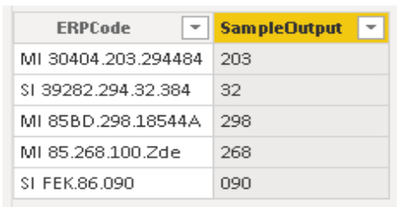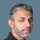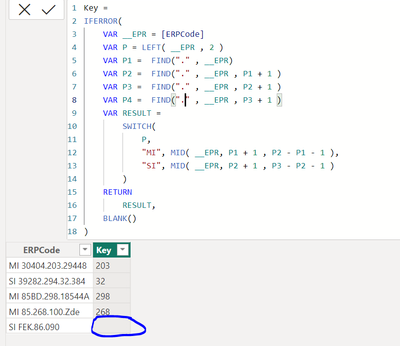cancel
Showing results for
Did you mean:Helper I

## DAX: Need help to find the 2nd, 3rd delimited values based on string

Assume we have the following column called [ERPCode] below. I want to dynamically return the second or third value within my serial key. If my ERP code contains "MI" return the second value, but if my ERP code contains "SI" then return me the third value in my serial key. A sample output is provided for reference.Thanks,

Mohan V.

1 ACCEPTED SOLUTIONCommunity Support

Hi @mohancsg ,

You just modify the dax formula provided by @Fowmy .

``````Column =
VAR _code = [ERPCode]
VAR _len =
LEN ( _code )
VAR _a =
FIND ( ".", _code )
VAR _b =
IFERROR ( FIND ( ".", _code, _a + 1 ), 0 )
VAR _c =
IFERROR ( FIND ( ".", _code, _b + 1 ), 0 )
VAR _val =
SWITCH (
TRUE (),
CONTAINSSTRING ( _code, "MI" ),
IF ( _b <> 0, MID ( _code, _a + 1, _b - _a - 1 ), MID ( _code, _a + 1, _len ) ),
CONTAINSSTRING ( _code, "SI" ),
IF ( _c <> 0, MID ( _code, _b + 1, _c - _b - 1 ), MID ( _code, _b + 1, _len ) )
)
RETURN
_val
``````Please refer the attached .pbix file.

Best regards,
Community Support Team_Binbin Yu
If this post helps, then please consider Accept it as the solution to help the other members find it more quickly.

5 REPLIES 5Community Support

Hi @mohancsg ,

You just modify the dax formula provided by @Fowmy .

``````Column =
VAR _code = [ERPCode]
VAR _len =
LEN ( _code )
VAR _a =
FIND ( ".", _code )
VAR _b =
IFERROR ( FIND ( ".", _code, _a + 1 ), 0 )
VAR _c =
IFERROR ( FIND ( ".", _code, _b + 1 ), 0 )
VAR _val =
SWITCH (
TRUE (),
CONTAINSSTRING ( _code, "MI" ),
IF ( _b <> 0, MID ( _code, _a + 1, _b - _a - 1 ), MID ( _code, _a + 1, _len ) ),
CONTAINSSTRING ( _code, "SI" ),
IF ( _c <> 0, MID ( _code, _b + 1, _c - _b - 1 ), MID ( _code, _b + 1, _len ) )
)
RETURN
_val
``````Please refer the attached .pbix file.

Best regards,
Community Support Team_Binbin Yu
If this post helps, then please consider Accept it as the solution to help the other members find it more quickly.Super User

@mohancsg

Will a Power Query solution work for you?

Did I answer your question? Mark my post as a solution! and hit thumbs upHelper I

@Fowmy No, I am looking for dax solution.Super User

@mohancsg

Here is the DAX solution, add this as a Calculated Column:

``````Key =
IFERROR(
VAR __EPR = [ERP Code]
VAR P = LEFT( __EPR , 2 )
VAR P1 =  FIND("." , __EPR)
VAR P2 =  FIND("." , __EPR , P1 + 1 )
VAR P3 =  FIND("." , __EPR , P2 + 1 )
VAR RESULT =
SWITCH(
P,
"MI", MID( __EPR, P1 + 1 , P2 - P1 - 1 ),
"SI", MID( __EPR, P2 + 1 , P3 - P2 - 1 )
)
RETURN
RESULT,
BLANK()
)
``````Did I answer your question? Mark my post as a solution! and hit thumbs upHelper I

@Fowmy It is working but only issue here is if i dont have any delimeter then it is returing blank as in below case.Announcements#### Exclusive opportunity for Women!

Join us for a free, hands-on Microsoft workshop led by women trainers for women where you will learn how to build a Dashboard in a Day!#### Power Platform Conference-Power BI and Fabric Sessions

Join us Oct 1 - 6 in Las Vegas for the Microsoft Power Platform Conference.Top Solution Authors
Top Kudoed Authors
Users online (1,861)